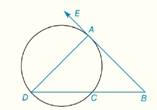Chapter 6.CR, Problem 6CRElementary Geometry For College St...

7th Edition
Alexander + 2 others
ISBN: 9781337614085

Solutions

Chapter
SectionElementary Geometry For College St...

7th Edition
Alexander + 2 others
ISBN: 9781337614085
Textbook Problem

In Review Exercises 5 to 10, B A → is tangent to the circle at point A in the figure shown. m A D C ^ = 295 ° ,     m A D ^ = 155 ° ,   m ∠ B =   ?To determine

To find: mB

Explanation

Theorem:

1) The measure of an angle formed when two secants intersect at a point outside the circle is one-half the difference of the measures of two intercepted arcs.

2) The measure of an inscribed angle of a circle is one-half the measure of its intercepted arc.

Calculation:

Given that

mADC^=295°, mAD^=155° and BA is tangent to the circle at point A.

But, mADC^+mAC^=360° since both the arcs completes the circle.

So, 295°+mAC^=360°

Subtract 295° from both the sides

295°+mAC^-295°=360°-295°

Therefore,

Still sussing out bartleby?

Check out a sample textbook solution.

See a sample solution

The Solution to Your Study Problems

Bartleby provides explanations to thousands of textbook problems written by our experts, many with advanced degrees!

Get Started

Test for divisibility by 2: 458

Elementary Technical Mathematics

Find the derivative. Simplify where possible. 31. f(x)=tanx

Single Variable Calculus: Early Transcendentals, Volume I

Divide the following fractions and reduce to lowest terms. 28.

Contemporary Mathematics for Business & Consumers

Simplify i. a. i b. -i c. 1 d. â€“l

Trigonometry (MindTap Course List)

Given that for all x, a power series for is:

Study Guide for Stewart's Single Variable Calculus: Early Transcendentals, 8th